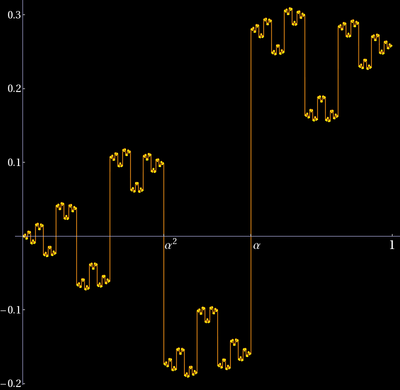# Golden graph project

In this project, (see [ArXiv]), we sum up correlated but identically distributed random variables Sn = X1 + ... + Xn which have a Cauchy distribution. They are examples of high risk stochastic process in the sense that where Xk are not in L1 but where E[1[-n,n] Xk] stays bounded. In other words, the Cauchy principal value of E[X] is finite but E[|X|] is infinity. Such processes model high volatility market situations, where the gain or loss Xk can be arbitrarily large. We also assume that E[1[-n,n]|X|k ] is of the order log(n) By Birkhoffs ergodic theorem (|X1| + ... + |Xn|)/n converges to infinity. The independent case is well understood since Sn is again Cauchy distributed. The central limit theorem looks different. What happens if the random variables are correlated? Surprisingly the random walk can become self similar. The golden graph which can be plotted quickly in Mathematica. Here is a large picture.Any such stochastic process can be obtained where T is a measure preserving automorphims of the interval [0,1] and Xk(x) = cot(pi x) + h(x) with a L1 function h. From the dynamical systems point of view, this is no restriction of generality since any automorphism of a Lebesgue probability space can be described by a measure preserving map of the circle [0,1] and any risky stochastic process with discrete time can be written as Xk = g(Tkx) for some function g.

The question whether Sn/n stays bounded or not depends very much on how fast the process approaches the singular set of g. We explore this in the simplest case, where T is a circle map on [0,1]. This looks very special but it is not. Even complicated Hamiltonian dynamical systems in finite or infinite dimensions have invariant sets on which the dynamics is conjugated to a group translation on a compact metric space. We also look at the situation, where the rotation number is furthest away from rational case, from resonances, which is the golden ratio. Also this is not as special as it seems since in real dynamical systems, this is the case which is the most robust and disappears last. Circle maps with golden rotation appear in nature since they are the most resilient subsystems under perturbations. Such motion occurs in realistic systems like nonlinear oscillators, particles trapped in a magnetic field.

Unlike in the case of a bounded stochastic process, where Birkhoffs ergodic theorem assures that Sn is C n, where C is the common expectation. The example under consideration is generated by a smooth circle map and a function which is continuous except at a single pole. We prove that the random walk Sn has a selfsimilar structure and the path converges, if rescaled so that the walk is always shown over the interval [0,1] the limit is the "golden graph". This is a new phenomenon for stochastic processes. It shows that that Sn scales nicely. This project addresses also an open problem raised in a project of 2008 with John Lesieutre and allows to prove observations found experimentally in the Spring 2010 with Folkert Tangerman

The problem is also related to the dependent random walk called curlicues: Assume you drive with constant speed on a straight line. After some fixed time T you turn in the direction of the sweep hand of the clock. Now drive again for the same time, then turn into the direction of the sweep hand etc. The traced path will be a curlicue in the limit.

There are other relations like in almost periodic discrete Schroedinger equations with cot potential which Barry Simon analyzed in the 80ties and called the Maryland model.

As mentioned in the papers, there are many connections with number theory. Actually, number theorists like Hardy and Littlewood where the first who have studied such random walks.

There are also relations with modular forms, the theory of integer partitions. The interplay between dynamical systems and number theory are fruitful since at least 100 years. A random walk studied by Hecke is just the imaginary part of the random walk I studied with Lesieutre and Tangerman.